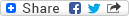#Hitro množenje matrik : delo diplomskega seminarja
ID Marinko, Matej (Avtor), ID Šivic, Klemen (Mentor) Več o mentorju...PDF - Predstavitvena datoteka, prenos (627,16 KB)MD5: E81A320D11990ABA4E12EB67A71C64F6

Izvleček
Množenje matrik je v linearni algebri preprosta operacija, ki se pogosto pojavlja v rešitvah najrazličnejših problemov. Prav zato je bilo v iskanje hitrih algoritmov za množenje matrik vloženega že veliko dela. V diplomskem delu definiramo problem iskanja zgornje meje eksponenta matričnega množenja in razvijemo teorijo ranga in mejnega ranga bilinearnih preslikav. Predstavimo več algoritmov za hitro množenje matrik, ki slonijo na tej teoriji. Izbrane algoritme tudi implementiramo, jih primerjamo med seboj in ocenimo njihovo uporabnost v praksi.

Jezik: Slovenski jezik množenje matrik, eksponent matričnega množenja, rang tenzorjev, mejni rang Diplomsko delo/naloga FMF - Fakulteta za matematiko in fiziko 2020 20.500.12556/RUL-120183004 5824435517.09.2020 620 137Kopiraj citat## Sekundarni jezik

Jezik: Angleški jezik Fast matrix multiplication Matrix multiplication is one of the most basic operations in linear algebra and thus very common in various scientific disciplines. Consequently, the computation complexity of matrix multiplication has been extensively studied. In this work, we define a problem of finding the upper bound for the exponent of matrix multiplication and present the theory of rank and border rank of bilinear maps. We describe multiple fast matrix multiplication algorithms based on this theory. In the end, we implement some selected algorithms, compare them, and discuss their value in practical applications. matrix multiplication, exponent of matrix multiplication, tensor rank, border rank

Nazaj